# Efficient way for finding Lower High ?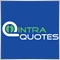11298

Hello Members,

Looking for a simpler logic to find Lower High under a certain time limit. Please see the picture for better understanding.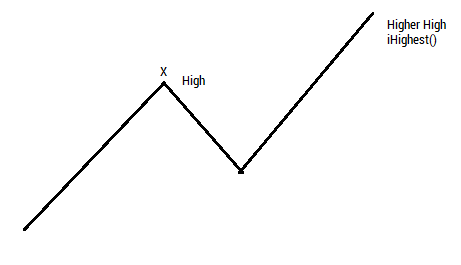In this case, I can find Highest point with iHighest Easily.

`Highest_barindex = iHighest(NULL,0,MODE_HIGH,80,1)`

But how to find the Lower High point X?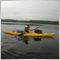993

You could use zigzag.

You could call the previous highest high starting from the most recent one and see if its value is higher.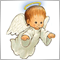3025

Somsri Sarkar:

Hello Members,

Looking for a simpler logic to find Lower High under a certain time limit. Please see the picture for better understanding.

In this case, I can find Highest point with iHighest Easily.

But how to find the Lower High point X?

You have to define a peak. E.g. a peak is a bar that has the highest price within x bars from the left and x bars from the right. Then you have to find out all peaks within the required interval and handle with them.20214

You found a high. Find the next low and the next high after that. Go down hill until you find the bottom, go up hill until you find the top.
```int     LocalExtremeBar(int length, int start, double d){ // d=direction= +1 or -1
if (d > 0)  return( LocalExtremeArray(High, length, start, d) );
return( LocalExtremeArray( Low, length, start, d) );
}
int     LocalExtremeArray(double arr[], int length, int iStart, double d){
while(true){
int iPrev = iStart;     iStart = ArrayExtrema(arr, length, iStart, d);
if (iStart == iPrev)    return iStart;
}
//NOTREACHED
}
int     ArrayExtrema(double arr[], int length, int iStart, double d){
int iExtreme = iStart;  double value = arr[iExtreme];
int iLimit = MathMinI(iStart + length, ArraySize(arr));
for(iStart++; iStart<iLimit; iStart++)  if((arr[iStart]-value) *d>= 0.){
iExtreme = iStart;  value = arr[iExtreme];  }   // largest index (>=)
return iExtreme;
}```3025

whroeder1:
You found a high. Find the next low and the next high after that. Go down hill until you find the bottom, go up hill until you find the top.

Hi, I'm just curious. Everybody has his own style. Be sure it isn't an attack. I wanted to ask you two questions.

1. Is there a reason to use: while(true){...;if(...) return ...;} instead of: do{...}while(...);return(...);
2. Is there a reason to use your ArrayExtrema() instead of ArrayMaximum()/ArrayMinimum()

I would write your code like something this (there could be bugs in my code, I haven't tested it, it's just an example of coding style):

```int     LocalExtremeBar(int length, int start, bool peak=true){ // peak or trough
if(peak) return( LocalExtremeArray(High, length, start) );
return( LocalExtremeArray( Low, length, start, peak) );
}
int     LocalExtremeArray(double &arr[], int length, int iStart, bool peak=true){
int iPrev;
do{
iPrev = iStart;
iStart = peak?ArrayMaximum(arr,length,iStart):ArrayMinimum(arr,length,iStart);
}
while(iStart != iPrev);
return iStart;
}```20214

Petr Nosek:
1. Is there a reason to use: while(true){...;if(...) return ...;} instead of: do{...}while(...);return(...);
2. Is there a reason to use your ArrayExtrema() instead of ArrayMaximum()/ArrayMinimum()

I would write your code like something this (there could be bugs in my code, I haven't tested it, it's just an example of coding style):

`int     LocalExtremeBar(int length, int start, bool peak=true){ // peak or trough`
1. while(true){ if(..) return} is the same as while(true){ if(...) break;} return; You can't use while(condition) because must execute the loop at least once. Code was written way before build 600 and there was no do{...}while(condition) which is what I would now use.

2. Could use built in functions except they don't specify what happens with double top (which index is returned.) I wanted to return the furthest (to start the next path.)

3. `if((arr[iStart]-value) *d>= 0.){`
You could but then you would have to rewrite the test for two directions, e.g.
```if( peek ? arr[iStart]-value) >= 0.
: arr[iStart]-value) <= 0.){```
My test is bidirectional. I write the code once, the buy case: Bid > signal. Then change to (Bid - signal) > 0 and then add the sell case is (Bid - signal) *d> 0.
Posted my code Why is there NO Complete EA within the Code-Base? - MQL4 and MetaTrader 4 - MQL4 programming forum It has not been adjusted for Build 600+.3025

whroeder1:
1. while(true){ if(..) return} is the same as while(true){ if(...) break;} return; You can't use while(condition) because must execute the list at least once. Code with writing way before build 600 and there was no do{...}while(condition) which is what I would now use.

2. Could use built in functions except they don't specify what happens with double top (which index is returned.) I wanted to return the furthest to start the next path.

3. You could but then you would have to rewrite the test for two directions, e.g. My test is bidirectional.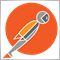2392

Best to use zigzag indicator via iCustom. Let the indicator thread handle ZZ calculations and just call to buffer. This is afterwards what op really wants.993

nicholishen:
Best to use zigzag indicator via iCustom. Let the indicator thread handle ZZ calculations and just call to buffer. This is afterwards what op really wants.
Easiest way probably11298

Hello Members,

Thank you so much for all of your suggestions & coding examples.

I first tried with the icustom Zigzag. With the following logic, I can find now the Lower High & also the Higher Lows. Here is my code.

```extern int div_period = 100;
double LH, HL;
/---
double HH = iHigh(NULL,0,iHighest(NULL,0,MODE_HIGH,div_period,0));
double LL = iLow(NULL,0,iLowest(NULL,0,MODE_HIGH,div_period,0));
// Find Lower High
for(int i = div_period; i>=0; i--)
{
double ZZ = iCustom(NULL,0,"ZigZag",0,i);
if(ZZ >0 && ZZ < HH && ZZ > LL && MathAbs(HH-ZZ) < MathAbs(LL-ZZ)){
LH = ZZ;
break;
}

}
// Finding Higher Low
for(int t = div_period; t>=0; t--)
{
double YY = iCustom(NULL,0,"ZigZag",0,t);
if(YY >0 && YY < HH && YY > LL && MathAbs(HH-YY) > MathAbs(LL-YY)){
HL = YY;
break;
}

}
printf(StringConcatenate("Highest: ",DoubleToStr(HH,Digits)," Lowest: ",DoubleToStr(LL,Digits)," LH: ",DoubleToStr(LH,Digits)," HL: ",DoubleToStr(HL,Digits)));```

Not sure it will work for the most the cases or not. But with picture example below it works.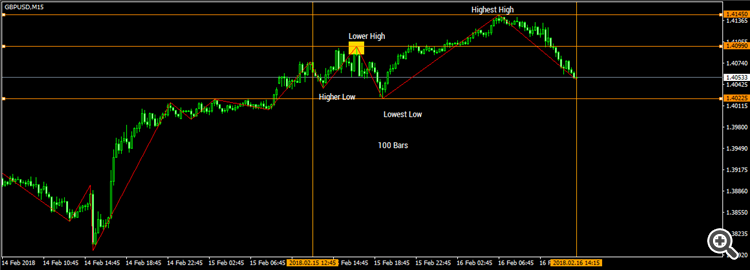The picture which I have posted earlier, in order to make such a perfect wave counting, we can add time element in this case & compare with time, i.e if the Lower High formed after the lowest low or not etc.

Is my coding logic is okay now?

Thank you

Regards